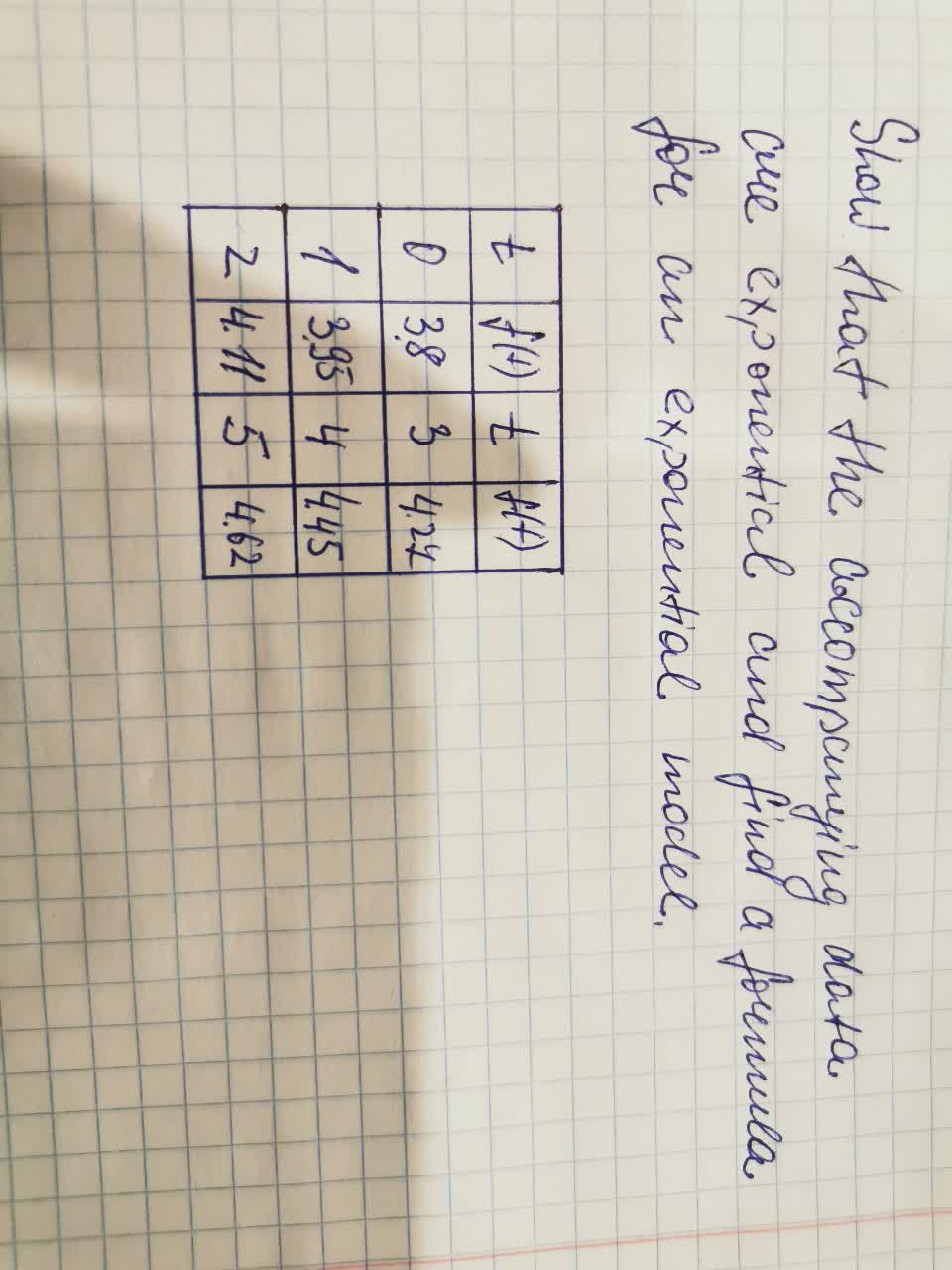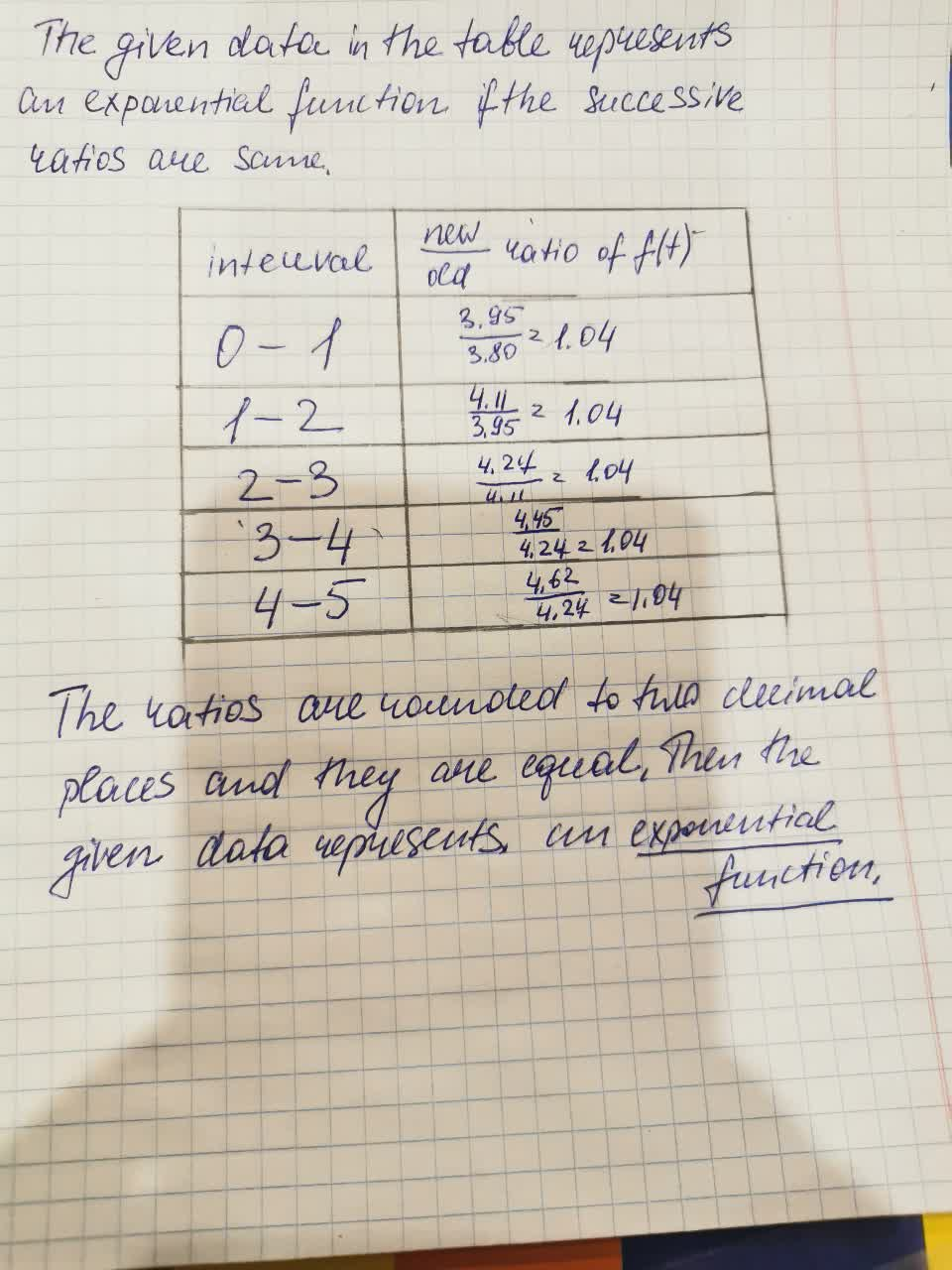QuestionExponential models
ANSWERED2021-08-12

1.2.Now we can see that the values of f(t) are increasing with are increment in the value of t. Thus the data represents an exponential growth function.
From the table we find that the initial value of the function is f(0)=3.80
And the growth factor is the successive ratio which is 1.04.
Now the exponential function is given by,
$$\displaystyle{f{{\left({t}\right)}}}={I}{n}{i}{t}{i}{a}{l}\ {v}{a}{l}{u}{e}\cdot{\left({G}{r}{o}{w}{t}{h}\ factor\right)}^{{t}}$$
i.e. $$\displaystyle{f{{\left({t}\right)}}}={3.80}\cdot{\left({1.04}\right)}^{{t}}$$